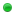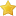Themabewertung:
• 0 Bewertung(en) - 0 im Durchschnitt
• 1
• 2
• 3
• 4
• 5
 DSM qualification - Instructions (English)HausigelHochhausfanatikerBeiträge: 668 Themen: 47 Registriert seit: Dec 2008 Bewertung: 2 07.03.2014, 12:44 Dear puzzle friends, in response to an inquiry from one of our Sudoku friends from abroad, I am posting a translation of the puzzle instructions. Please note that this is only an unofficial attempt to translate the rules; if you have any questions, please ask one of the officials. --- General information: The contest consists of 18 puzzles, the total solving time is 120 minutes (no overtime). In each puzzle, the answer key consists of two rows; enter all digits within those two rows from left to right (first the upper one, then the lower one), including all the givens. The points for each puzzle can be found on page 1 of the German instruction file; there will be no penalty for wrong entries. A brief translation of the rules of all variants in this contest follows. Please note that the order of the variants is not the same as the order of the contest puzzles. --- Standard Sudoku (puzzles 1, 11, 17 and 18): Enter the numbers 1 to 9 in the grid such that each number occurs exactly once in every row, column and outlined region. (Unless explicitly stated otherwise, these rules also apply to all variants in this contest.) Diagonal Sudoku (puzzle 2): Each number must occur exactly once on each of the two main diagonals as well. Killer Sudoku (puzzles 3 and 16): The grid has been divided into cages. The sum of the numbers within each cage is given. Numbers cannot repeat within a cage. Größer Kleiner Gleich Sudoku (puzzle 4): Each arrow points from a larger to a smaller number. A line without an arrow indicates that both numbers are the same. Ungerade Sudoku (puzzle 5): All the grey cells must contain odd numbers. Acht bilden Sudoku (puzzle 6): Whenever an edge between two adjacent cells contains a dot, the number 8 can be obtained from the two numbers as the result of an arithmetic operation (addition, subtraction or multiplication). All possible dots are given, i.e. if there is no dot, it is not possible to obtain the result of 8 this way. Pfeile Sudoku (puzzle 7): Arrows have been placed inside the grid which cover three cells each. The number at the tip of the arrow is equal to the sum of the other two numbers. Circle Sudoku (puzzle 8): Circles have been placed inside the grid which touch four cells each. The four numbers given in the circle must be equal to the four numbers in the adjacent cells in the same order; however, the circles can be rotated. Addition Sudoku (puzzle 9): The number in each circle is equal to the sum of the numbers along the respective line. Oddmax Sudoku (puzzle 10): Circle which contain numbers have been placed on some vertices of the grid. The number inside the circle must occur in exactly one of the four neighboring cells, and it must be the highest odd number within those four cells. 9 Dragons Sudoku (puzzle 12): Lines which cover nine cells each have been drawn in the grid. Along each such line, every number from 1 to 9 must occur exactly once as well. Pünktchen Sudoku (puzzle 13): Whenever an edge between two adjacent cells contains a dot, the difference of the two respective numbers must be 1. All possible dots are given, i.e. if there is no dot, the difference of the two numbers must be higher than 1. Nachbarn Neun Sudoku (puzzle 14): The numbers outside the grid indicate which numbers are adjacent to the 9 in the respective row or column. (A two-digit number means that both its digits are adjacent to the 9.) 9er Suche Sudoku (puzzle 15): Each arrow points to the number 9 in the same row or column; the digit inside the arrow cell indicates the distance from the arrow cell to the respective cell with the number 9. --- Enjoy the puzzles! Hausigel

Gehe zu:

Benutzer, die gerade dieses Thema anschauen: 1 Gast/Gäste